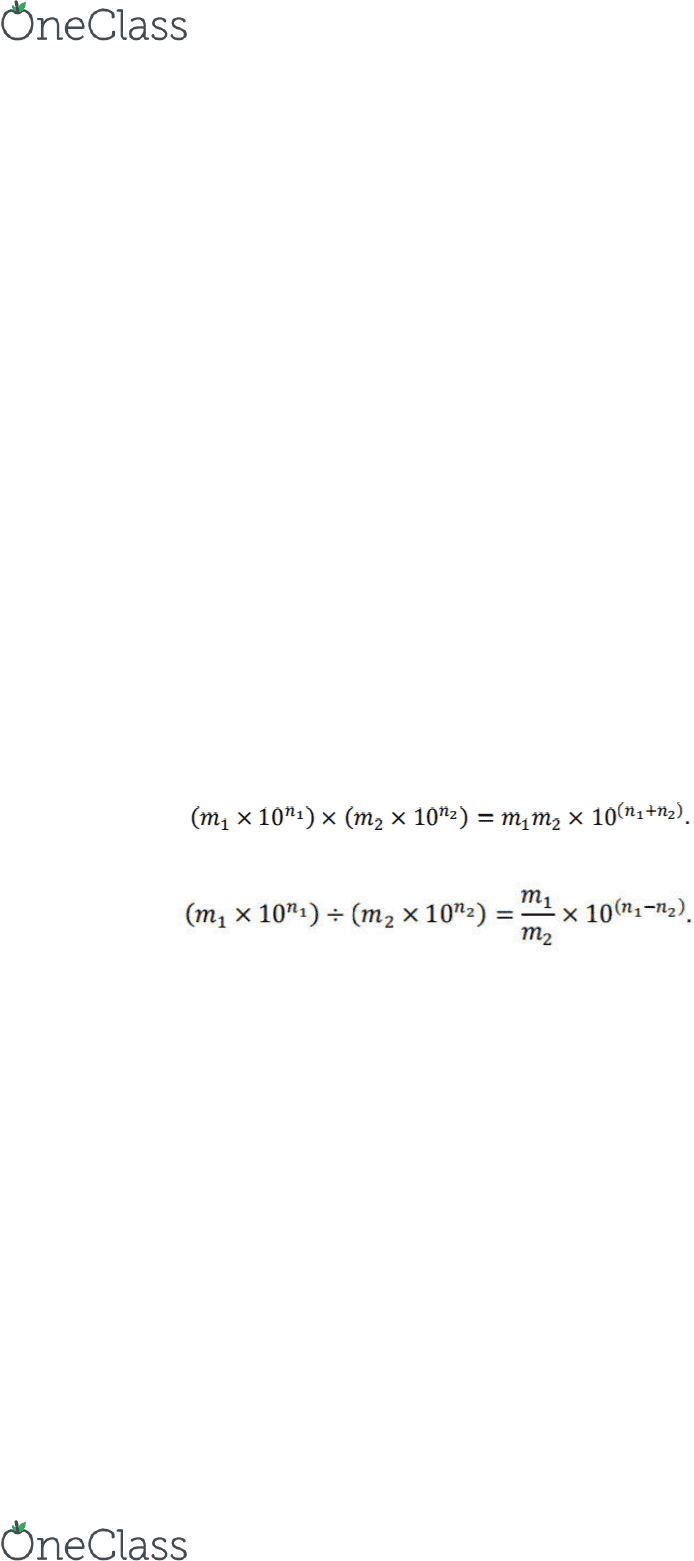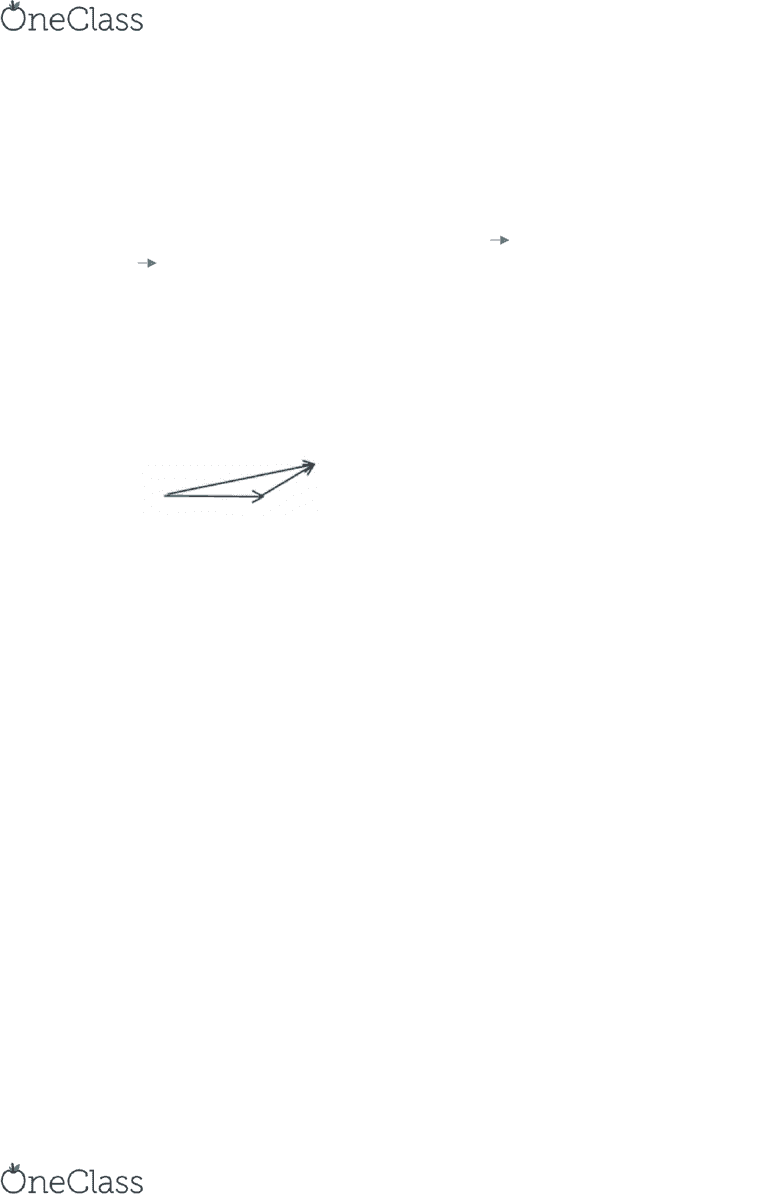Study Guides (390,000)
US (220,000)
UF (3,000)
PHY (400)
Study Guide

# PHY 2020- Final Exam Guide - Comprehensive Notes for the exam ( 31 pages long!)

Department
Physics
Course Code
PHY 2020
Professor
Sergei Obukhov
Study Guide
Final

This preview shows pages 1-3. to view the full 31 pages of the document.UF
PHY 2020
FINAL EXAM
STUDY GUIDE

Only pages 1-3 are available for preview. Some parts have been intentionally blurred.Only pages 1-3 are available for preview. Some parts have been intentionally blurred.1.1:
Physics is the science of matter and energy. (Even though matter is a form of energy)
Physics is an experimental science (based on experimental results).
We do an experiment and try and understand its results, and try and think of another
experiment to confirm them or not.
Theorists predict the results. Experimentalists do the experiments and find results.
Theorists interpret those results and find out what they mean (not all experiment results
can be proved).
Scientific theories have three major components: (1) The theory must match all relevant
experimental data - theorists can't pick and choose data to match! (2) Theories must be
able to make predictions. (3) Theories should be able to be disproved - this is also known
as falsifiability, so we can test whether they are correct or not. Note, theories are not
required to make large advancements in knowledge; often, science advances in many
small steps building on previous findings from other scientists. In this way, it is a very
collaborative endeavor!
A theory that has been tested and proven correct over and over again, it is written down
and called a law (this doesn’t necessarily mean it’s 100% correct).
1.2:
Scientific notation is crucial in Physics.
Multiplying two numbers in scientific notation is written in the form
Dividing two numbers in scientific notation is written in the form
1.3:
Standard International System of Units (SI Units): meter (for length), kilogram (for
mass), mass (for time).
2.54 cm = 1 inch **** KNOW THIS
Always make sure to check your units after doing conversions, and to check that the
find more resources at oneclass.com
find more resources at oneclass.com

Unlock to view full version

Only pages 1-3 are available for preview. Some parts have been intentionally blurred.2.1:
Scalar quantities have no direction attached, but have a magnitude (size). Ex: mass
Vector quantities have a direction and a magnitude. Ex: velocity
A vector is often showed as an arrow. The direction tells us the direction of the vector,
and the length of it tells us the magnitude.
All vectors have an arrow above their label: Ex: A
|A| = A (magnitude of A)
Adding scalars is easy, but adding vectors is more difficult (as we need to define
direction as well as magnitude).
If you have an equation, it has to be completely vector or completely scalar (each side of
the equals sign can’t be different.
When adding vectors, connect one vector (A) to the end of another (B), and then draw a
third vector connecting the two. The third side of the “triangle” is vector C (if A + B =
C).
For this, we still won’t know exactly what C equals, but we
have a better approximation.
The only time we can actually add them if the vectors are exactly opposites in direction,
or exactly the same direction, or if one vector is horizontal, and another is vertical (we
can make a right triangle).
2.2:
sin = opp/hyp
Understand how to do right angle trigonometry.
2.3:
You can use x and y components to add vectors, as long as you know the vector’s
magnitude and its angle (or have enough information to determine those using
trigonometry).
When trying to find the sum of vectors with z-components, add all components
separately. Then use Pythagorean theorem.
o Ex: The c omp o ne nts of a vector V can be writte n a s ( V_x, V_y, V_z). Wh a t
is the le n g th of a ve ctor which is th e s um of th e two ve ctors V_1 with
components (5.0, -4.8, -3.3)a n d V_2 with compo n e n ts (7.8, -5.1, 0.0)?
Firstly a dd the x, y a nd z compone nts of the two ve ctors V_1 a nd V_2 to ge t the
compone nts of the re s ulta nt vector to be (12.8, -9.9, -3.3). The length of this
vector would be sqrt [(12.8)^2 + (-9.9)^2 + (-3.3)^2] = sqrt(272.74).
find more resources at oneclass.com
find more resources at oneclass.com